International
Tables for
Crystallography
Volume C
Mathematical, physical and chemical tables
Edited by E. Prince

International Tables for Crystallography (2006). Vol. C, ch. 2.2, pp. 26-29

## Section 2.2.1. Laue geometry

J. R. Helliwella

aDepartment of Chemistry, University of Manchester, Manchester M13 9PL, England

### 2.2.1. Laue geometry

| top | pdf |

The main book dealing with Laue geometry is Amorós, Buerger & Amorós (1975). This should be used in conjunction with Henry, Lipson & Wooster (1951), or McKie & McKie (1986); see also Helliwell (1992, chapter 7). There is a synergy between synchrotron and neutron Laue diffraction developments (see Helliwell & Wilkinson, 1994).

#### 2.2.1.1. General

| top | pdf |

The single crystal is bathed in a polychromatic beam of X-rays containing wavelengths between λmin and λmax. A particular crystal plane will pick out a general wavelength λ for which constructive interference occurs and reflect according to Bragg's law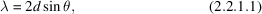where d is the interplanar spacing andis the angle of reflection. A sphere drawn with radius 1/λ and with the beam direction as diameter, passing through the origin of the reciprocal lattice (the point O in Fig. 2.2.1.1) , will yield a reflection in the direction drawn from the centre of the sphere and out through the reciprocal-lattice point (relp) provided the relp in question lies on the surface of the sphere. This sphere is known as the Ewald sphere. Fig. 2.2.1.1shows the Laue geometry, in which there exists a nest of Ewald spheres of radii between 1/λmax and 1/λmin. An alternative convention is feasible whereby only a single Ewald sphere is drawn of radius 1 reciprocal-lattice unit (r.l.u.). Then each relp is no longer a point but a streak between λmin/d and λmax/d from the origin of reciprocal space (see McKie & McKie, 1986, p. 297). In the following discussions on the Laue approach, this notation is not followed. We use the nest of Ewald spheres of varying radii instead.Figure 2.2.1.1 | top | pdf |Laue geometry. A polychromatic beam containing wavelengths λmin to λmax impinges on the crystal sample. The resolution sphere of radius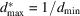is drawn centred at O, the origin of reciprocal space. Any reciprocal-lattice point falling in the shaded region is stimulated. In this diagram, the radius of each Ewald sphere uses the convention 1/λ.

Any relp (hkl) lying in the region of reciprocal space between the 1/λmax and 1/λmin Ewald spheres and the resolution sphere 1/dmin will diffract (the shaded area in Fig. 2.2.1.1). This region of reciprocal space is referred to as the accessible or stimulated region. Fig. 2.2.1.2shows a predicted Laue pattern from a well aligned protein crystal. For a description of the indexing of a Laue photograph, see Bragg (1928, pp. 28, 29).Figure 2.2.1.2 | top | pdf |A predicted Laue pattern of a protein crystal with a zone axis parallel to the incident, polychromatic X-ray beam. There is a pronounced blank region at the centre of the film (see Subsection 2.2.1.2). The spot marked N is one example of a nodal spot (see Subsection 2.2.1.4).

For a Laue spot at a given, only the ratio λ/d is determined, whether it is a single or a multiple relp component spot. If the unit-cell parameters are known from a monochromatic experiment, then a Laue spot at a givenyields λ since d is then known. Conversely, precise unit-cell lengths cannot be determined from a Laue pattern alone; methods are, however, being developed to determine these (see Carr, Cruickshank & Harding, 1992).

The maximum Bragg angle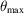is given by the equation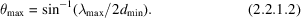#### 2.2.1.2. Crystal setting

| top | pdf |

The main use of Laue photography has in the past been for adjustment of the crystal to a desired orientation. With small-molecule crystals, the number of diffraction spots on a monochromatic photograph from a stationary crystal is very small. With unfiltered, polychromatic radiation, many more spots are observed and so the Laue photograph serves to give a better idea of the crystal orientation and setting prior to precession photography. With protein crystals, the monochromatic still is used for this purpose before data collection via an area detector. This is because the number of diffraction spots is large on a monochromatic still and in a protein-crystal Laue photograph the stimulated spots from the Bremsstrahlung continuum are generally very weak. Synchrotron-radiation Laue photographs of protein crystals can be recorded with short exposure times. These patterns consist of a large number of diffraction spots.

Crystal setting via Laue photography usually involves trying to direct the X-ray beam along a zone axis. Angular mis-setting anglesin the spindle and arc are easily calculated from the formula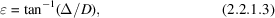where Δ is the distance (resolved into vertical and horizontal) from the beam centre to the centre of a circle of spots defining a zone axis and D is the crystal-to-film distance.

After suitable angular correction to the sample orientation, the Laue photograph will show a pronounced blank region at the centre of the film (see Fig. 2.2.1.2). This radius of the blank region is determined by the minimum wavelength in the beam and the magnitude of the reciprocal-lattice spacing parallel to the X-ray beam (see Jeffery, 1958). For the case, for example, of the X-ray beam perpendicular to the a*b* plane, then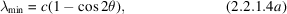where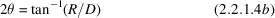and R is the radius of the blank region (see Fig. 2.2.1.2), and D is the crystal-to-flat-film distance. If λmin is known then an approximate value of c, for example, can be estimated. The principal zone axes will give the largest radii for the central blank region.

#### 2.2.1.3. Single-order and multiple-order reflections

| top | pdf |

In Laue geometry, several relp's can occur in a Laue spot or ray. The number of relp's in a given spot is called the multiplicity of the spot. The number of spots of a given multiplicity can be plotted as a histogram. This is known as the multiplicity distribution. The form of this distribution is dependent on the ratio λmaxmin. The multiplicity distribution in Laue diffraction is considered in detail by Cruickshank, Helliwell & Moffat (1987).

Any relp nh, nk, nl (n integer) will be stimulated by a wavelength λ/n since dnhnknl = dhkl/n, i.e.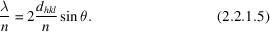However, dnhnknl must be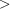dmin as otherwise the reflection is beyond the sample resolution limit.

If h, k, l have no common integer divisor and if 2h, 2k, 2l is beyond the resolution limit, then the spot on the Laue diffraction photograph is a single-wavelength spot. The probability that h, k, l have no common integer divisor is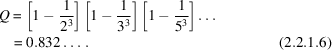Hence, for a relp where dmindhkl2dmin there is a very high probability (83.2%) that the Laue spot will be recorded as a single-wavelength spot. Since this region of reciprocal space corresponds to 87.5% (i.e. 7/8) of the volume of reciprocal space within the resolution sphere then 0.875 × 0.832 = 72.8% is the probability for a relp to be recorded in a single-wavelength spot. According to W. L. Bragg, all Laue spots should be multiple. He reasoned that for each h, k, l there will always be a 2h, 2k, 2l etc. lying within the same Laue spot. However, as the resolution limit is increased to accommodate this many more relp's are added, for which their hkl's have no common divisor.

The above discussion holds for infinite bandwidth. The effect of a more experimentally realistic bandwidth is to increase the proportion of single-wavelength spots.

The number of relp's within the resolution sphere is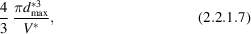where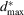= 1/dmin and V* is the reciprocal unit-cell volume.

The number of relp's within the wavelength band λmax to λmin, for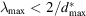, is (Moffat, Schildkamp, Bilderback & Volz, 1986)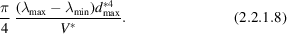Note that the number of relp's stimulated in a 0.1 Å wavelength interval, for example between 0.1 and 0.2 Å, is the same as that between 1.1 and 1.2 Å, for example. A large number of relp's are stimulated at one orientation of the crystal sample.

The proportion of relp's within a sphere of small d* (i.e. at low resolution) actually stimulated is small. In addition, the probability of them being single is zero in the infinite-band-width case and small in the finite-bandwidth case. However, Laue geometry is an efficient way of measuring a large number of relp's betweenand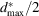as single-wavelength spots.

The above is a brief description of the overall multiplicity distribution. For a given relp, even of simple hkl values, lying on a ray of several relp's (multiples of hkl), a suitable choice of crystal orientation can yield a single-wavelength spot. Consider, for example, a spot of multiplicity 5. The outermost relp can be recorded at long wavelength with the inner relp's on the ray excluded since they need λ's greater than λmax (Fig. 2.2.1.3). Alternatively, by rotating the sample, the innermost relp can be measured uniquely at short wavelength with the outer relp's excluded (they require λ's shorter than λmin). Hence, in Laue geometry several orientations are needed to recover virtually all relp's as singles. The multiplicity distribution is shown in Fig. 2.2.1.4as a function of λmaxmin (with the corresponding values of δλ/λmean).Figure 2.2.1.3 | top | pdf |A multiple component spot in Laue geometry. A ray of multiplicity 5 is shown as an example. The inner point A corresponds to d and a wavelength λ, the next point, B, is d/2 and wavelength λ/2. The outer point E corresponds to d/5 and λ/5. Rotation of the sample will either exclude inner points (at the λmax surface) or outer points (at the λmin surface) and so determine the recorded multiplicity.Figure 2.2.1.4 | top | pdf |The variation with M = λmax/λmin of the proportions of relp's lying on single, double, and triple rays for the case. From Cruickshank, Helliwell & Moffat (1987).

#### 2.2.1.4. Angular distribution of reflections in Laue diffraction

| top | pdf |

There is an interesting variation in the angular separations of Laue reflections that shows up in the spatial distributions of spots on a detector plane (Cruickshank, Helliwell & Moffat, 1991). There are two main aspects to this distribution, which are general and local. The general aspects refer to the diffraction pattern as a whole and the local aspects to reflections in a particular zone of diffraction spots.

The general features include the following. The spatial density of spots is everywhere proportional to 1/D2, where D is the crystal-to-detector distance, and to 1/V*, where V* is the reciprocal-cell volume. There is also though a substantial variation in spatial density with diffraction angle; a prominent maximum occurs at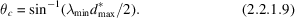Local aspects of these patterns particularly include the prominent conics on which Laue reflections lie. That is, the local spatial distribution is inherently one-dimensional in character. Between multiple reflections (nodals), there is always at least one single and therefore nodals have a larger angular separation from their nearest neighbours. The blank area around a nodal in a Laue pattern (Fig. 2.2.1.2) has been noted by Jeffery (1958). The smallest angular separations, and therefore spatially overlapped cases, are associated with single Laue reflections. Thus, the reflections involved in energy overlaps – the multiples – form a set largely distinct, except at short crystal-to-detector distances, from those involved in spatial overlaps, which are mostly singles (Helliwell, 1985).

From a knowledge of the form of the angular distribution, it is possible, e.g. from the gaps bordering conics, to estimateand λmin. However, a development of this involving gnomonic projections can be even more effective (Cruickshank, Carr & Harding, 1992).

#### 2.2.1.5. Gnomonic and stereographic transformations

| top | pdf |

A useful means of transformation of the flat-film Laue pattern is the gnomonic projection. This converts the pattern of spots lying on curved arcs to points lying on straight lines. The stereographic projection is also used. Fig. 2.2.1.5shows the graphical relationships involved [taken from International Tables, Vol. II (Evans & Lonsdale, 1959)], for the case of a Laue pattern recorded on a plane film, between the incident-beam direction SN, which is perpendicular to a film plane and the Laue spot L and its spherical, stereographic, and gnomonic points Sp, St and G and the stereographic projection Sr of the reflected beams. If the radius of the sphere of projection is taken equal to D, the crystal-to-film distance, then the planes of the gnomonic projection and of the film coincide. The lines producing the various projection poles for any given crystal plane are coplanar with the incident and reflected beams. The transformation equations are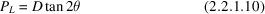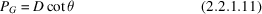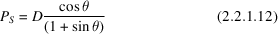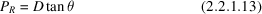Figure 2.2.1.5 | top | pdf |Geometrical principles of the spherical, stereographic, gnomonic, and Laue projections. From Evans & Lonsdale (1959).

### References

Amorós, J. L., Buerger, M. J. & Amorós, M. C. (1975). The Laue method. New York: Academic Press.Google Scholar
Bragg, W. H. (1928). An introduction to crystal structure analysis. London: Bell.Google Scholar
Carr, P. D., Cruickshank, D. W. J. & Harding, M. M. (1992). The determination of unit-cell parameters from Laue diffraction patterns using their gnomonic projections. J. Appl. Cryst. 25, 294–308.Google Scholar
Cruickshank, D. W. J., Carr, P. D. & Harding, M. M. (1992). Estimation of dmin, λmin and λmax from the gnomonic projections of Laue patterns. J. Appl. Cryst. 25, 285–293.Google Scholar
Cruickshank, D. W. J., Helliwell, J. R. & Moffat, K. (1987). Multiplicity distribution of reflections in Laue diffraction. Acta Cryst. A43, 656–674.Google Scholar
Cruickshank, D. W. J., Helliwell, J. R. & Moffat, K. (1991). Angular distribution of reflections in Laue diffraction. Acta Cryst. A47, 352–373.Google Scholar
Evans, H. T. & Lonsdale, K. (1959). Diffraction geometry. International tables for X-ray crystallography, Vol. II, p. 164. Birmingham: Kynoch Press.Google Scholar
Helliwell, J. R. (1985). Protein crystallography with synchrotron radiation. J. Mol. Struct. 130, 63–91.Google Scholar
Helliwell, J. R. (1992). Macromolecular crystallography with synchrotron radiation. Cambridge University Press.Google Scholar
Helliwell, J. R. & Wilkinson, C. (1994). X-ray and neutron Laue diffraction. In Neutron and synchrotron radiation for condensed matter studies: applications to soft condensed matter and biology, Vol. III, edited by J. Baruchel, J. L. Hodeau, M. S. Lehmann, J. R. Regnard & C. Schlenker. Berlin: Springer Verlag.Google Scholar
Henry, N. F. M., Lipson, H. & Wooster, W. A. (1951). The interpretation of X-ray diffraction photographs. London: Macmillan.Google Scholar
International Tables for X-ray Crystallography (1959). Vol. II. Birmingham: Kynoch Press.Google Scholar
Jeffery, J. W. (1958). An investigation of the blank areas on Laue photographs round: 1. The direct beam, and 2. Reflections with simple indices. Z. Kristallogr. 110, 321–328.Google Scholar
McKie, D. & McKie, C. (1986). Essentials of crystallography. Oxford: Blackwell Scientific Publications.Google Scholar
Moffat, K., Schildkamp, W., Bilderback, D. H. & Volz, K. (1986). Laue diffraction from biological samples. Nucl. Instrum. Methods, A246, 617–623.Google Scholar